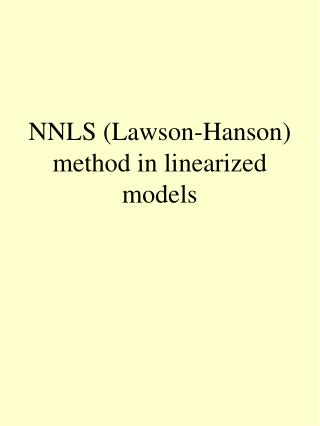# NNLS (Lawson-Hanson) method in linearized models - PowerPoint PPT PresentationDownload PresentationNNLS (Lawson-Hanson) method in linearized models

NNLS (Lawson-Hanson) method in linearized models
Download Presentation## NNLS (Lawson-Hanson) method in linearized models

- - - - - - - - - - - - - - - - - - - - - - - - - - - E N D - - - - - - - - - - - - - - - - - - - - - - - - - - -
##### Presentation Transcript

1. NNLS (Lawson-Hanson) method in linearized models

2. LSI & NNLS • LSI = Least square with linear equality constraints • NNLS = nonnegative least square

3. Flowchart

4. Initial conditions • Sets Z and P • Variables indexed in the set Z are held at value zero • Variables indexed in the set P are free to take values different from zero • Initially and P:=NULL

5. Flowchart

6. Stopping condition • Start of the main loop • Dual vector • Stopping condition: set Z is empty or

7. Flowchart

8. Manipulate indexes • Based on dual vector, one parameter indexed in Z is chosen to be estimated • Index of this parameter is moved from set Z to set P

9. Flowchart

10. Compute subproblem • Start of the inner loop • Subproblem where column j of Ep

11. Flowchart

12. Nonnegativity conditions • If z satisfies nonnegativity conditions then we set x:=z and jump to stopping condition • else continue

13. Flowchart

14. Manipulating the solution • x is moved towards z so that every parameter estimate stays positive. Indexes of estimates that are zero are moved from P to Z. The new subproblem is solved.

15. Testing the algorithm • Ex. Values of polynomial are calculated at points x=1,2,3,4 with fixed p1 and p2. • Columns of E hold the values of polynomial y(x)=x and polynomial at points x=1,2,3,4. • Values of p1and p2 are estimated with NNLS.

16. nnls_test 0.1 (c) 2003 by Turku PET Centre Matrix E: 1 1 2 4 3 9 4 16 Vector f: 0.6 2.2 4.8 8.4 Result vector:0.1 0.5

17. nnls_test 0.1 (c) 2003 by Turku PET Centre Matrix E: 1 1 1 2 4 8 3 9 27 4 16 64 Vector f: 0.73 3.24 8.31 16.72 Result vector:0.1 0.5 0.13

18. nnls_test 0.1 (c) 2003 by Turku PET Centre Matrix E: 1 1 1 1 2 4 8 16 3 9 27 81 4 16 64 256 Vector f: 0.73 3.24 8.31 16.72 Result vector:0.1 0.5 0.13 0

19. nnls_test 0.1 (c) 2003 by Turku PET Centre Matrix E: 1 1 1 2 4 8 3 9 27 4 16 64 Vector f: 0.23 1.24 3.81 8.72 Result vector:0.1 7.26423e-16 0.13

20. Kaisa Sederholm: Turku PET Centre Modelling report TPCMOD0020 2003-05-23